Question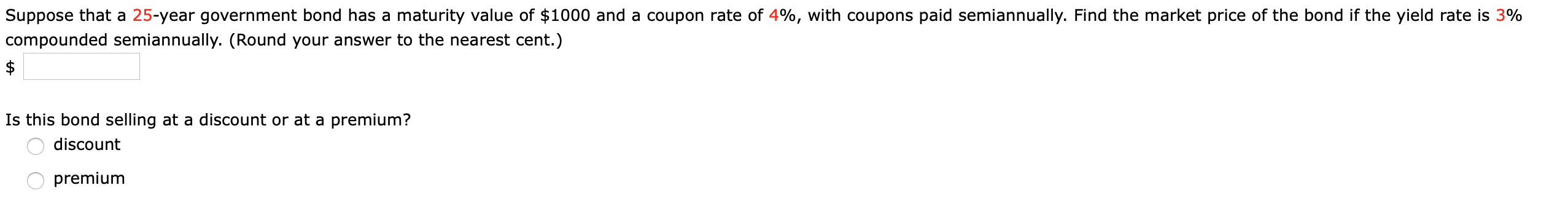As Bond is Semiannual

Coupon Rate = 4%/2 = 2%

YTM = 3%/2 = 1.5%

Period = 25*2 = 50

Bond Price = Interest*PVIFA(1.5%,50) + Par Value*PVIF(1.5%,50)

= 20 * 34.9997 + 1000*0.4750

Bond Price = \$1,174.99 or \$1,175

Since, it is more than Maturity Value(\$1000) by \$175, So Bond is at Premium.

#### Earn Coins

Coins can be redeemed for fabulous gifts.

Similar Homework Help Questions
• ### 5. -/1 points HarMathAp 12 6.4.011. My Notes Ask Your Teacher Suppose that a 25-year government...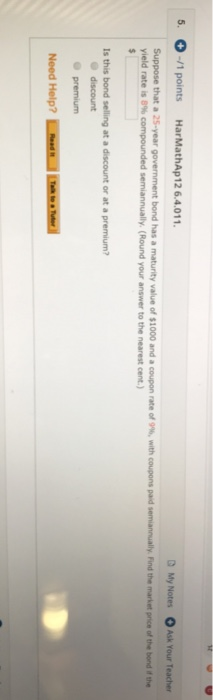5. -/1 points HarMathAp 12 6.4.011. My Notes Ask Your Teacher Suppose that a 25-year government bond has a maturity value of \$1000 and a coupon rate of 9%, with coupons paid semiannually. Find the market price of the bond if the yield rate is 8% compounded semiannually. (Round your answer to the nearest cent.) Is this bond selling at a discount or at a premium? discount premium Need Help?

• ### The yield to maturity of a \$1,000 bond with a 7.4% coupon rate, semiannual coupons, and...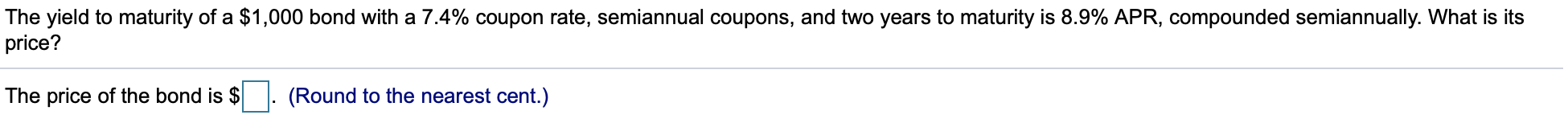The yield to maturity of a \$1,000 bond with a 7.4% coupon rate, semiannual coupons, and two years to maturity is 8.9% APR, compounded semiannually. What is its price? The price of the bond is \$ . (Round to the nearest cent.) Suppose a five-year, \$1,000 bond with annual coupons has a price of \$901.23 and a yield to maturity of 5.9%. What is the bond's coupon rate? The bond's coupon rate is %. (Round to three decimal places.)

• ### 25-year bond has a \$1,000 face value, a 10% yield to maturity, and an 8% annual...

25-year bond has a \$1,000 face value, a 10% yield to maturity, and an 8% annual coupon rate, paid semi-annually. What is the market value of the bond? Suppose a bond with a 10% coupon rate and semiannual coupons, has a face value of \$1000, 20 years to maturity and is selling for \$1197.93. What’s the YTM?

• ### The yield to maturity of a \$1000 bond with a 6.7% coupon rate, semiannual coupons, and...

The yield to maturity of a \$1000 bond with a 6.7% coupon rate, semiannual coupons, and two years to maturity is 7.6% APR, compounded semiannually, what is the price?

• ### A coupon bond with a par value of \$1,000 and a 10-year maturity pays semiannual coupons...

A coupon bond with a par value of \$1,000 and a 10-year maturity pays semiannual coupons of \$21. (a) Suppose the yield for this bond is 4% per year compounded semiannually. What is the price of the bond? (b) Is the bond selling above or below par value? Why?

• ### PROBLEM 2 A/ Suppose a ten-year, \$1,000 bond with an 8.8% coupon rate and semiannual coupons...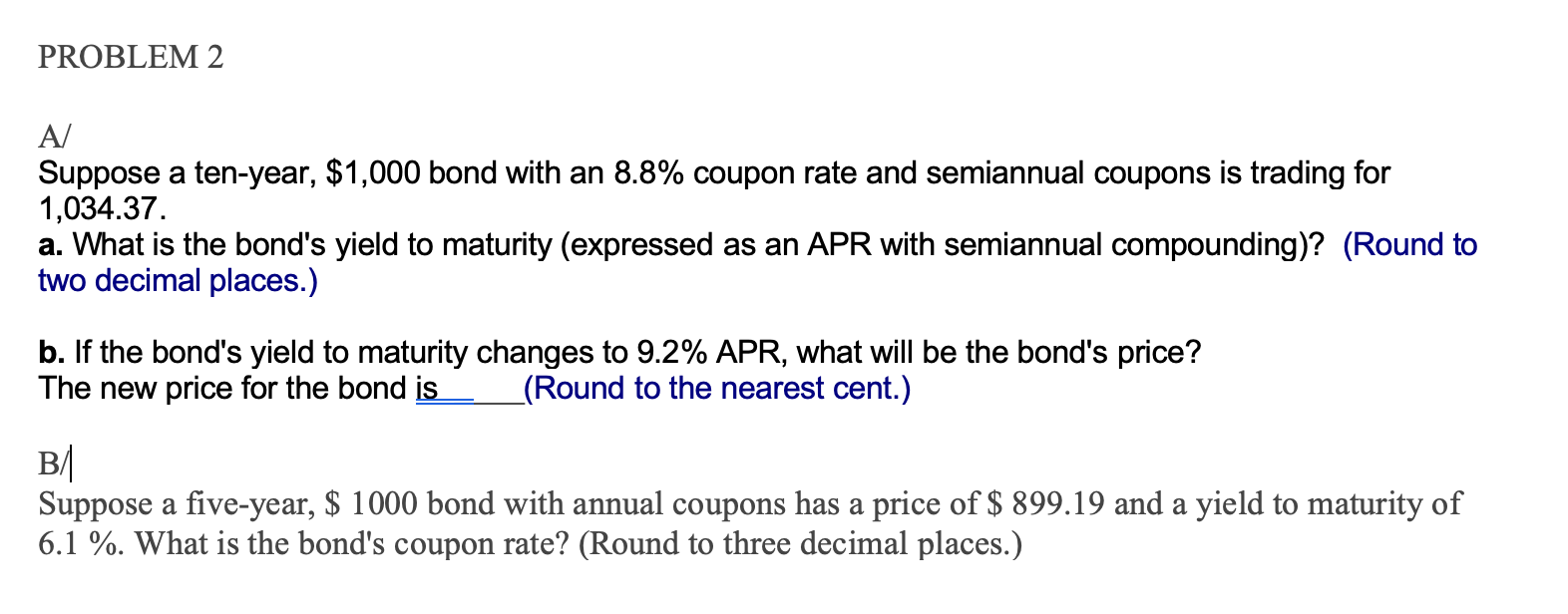PROBLEM 2 A/ Suppose a ten-year, \$1,000 bond with an 8.8% coupon rate and semiannual coupons is trading for 1,034.37. a. What is the bond's yield to maturity (expressed as an APR with semiannual compounding)? (Round to two decimal places.) b. If the bond's yield to maturity changes to 9.2% APR, what will be the bond's price? The new price for the bond is (Round to the nearest cent.) B/ Suppose a five-year, \$ 1000 bond with annual coupons has...

• ### A bond that has a face value of \$2,500 and coupon rate of 4.80% payable semi-annually...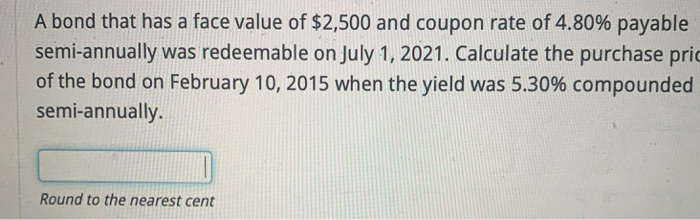A bond that has a face value of \$2,500 and coupon rate of 4.80% payable semi-annually was redeemable on July 1, 2021. Calculate the purchase pric of the bond on February 10, 2015 when the yield was 5.30% compounded semi-annually. Round to the nearest cent A \$8,000 bond that carries a 3.50% coupon rate payable semi-annually is purchased 6 years before maturity when the yield rate was 4.50% compounded semi-annually. a. Calculate the purchase price of the bond. \$0.00 Round...

• ### 5. A 30-year 1000 par value bond with coupons at 9% payable semiannually and a redemption...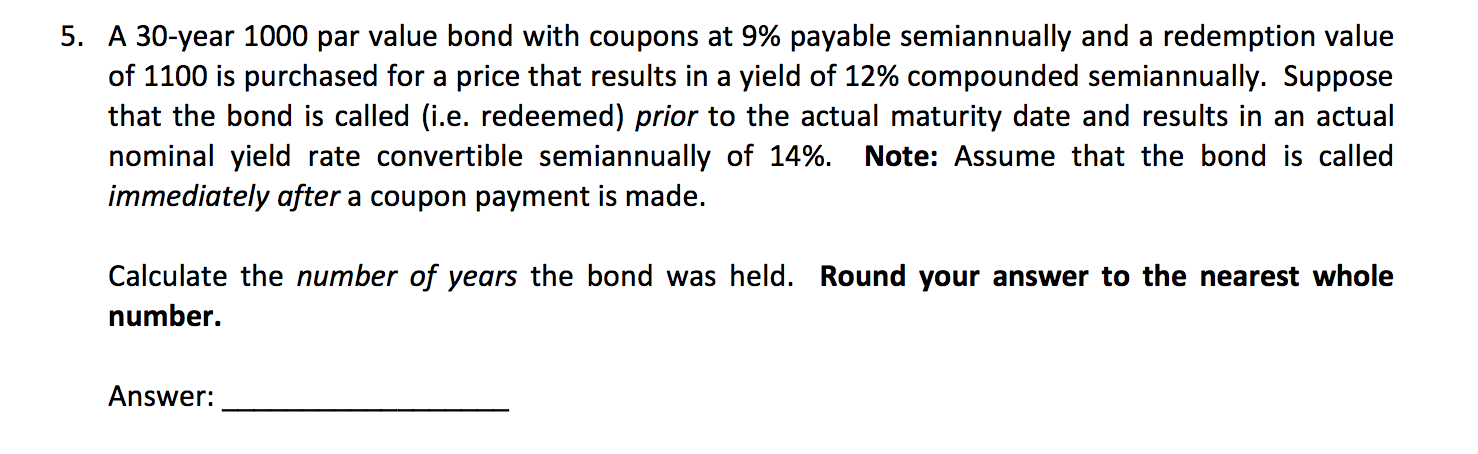5. A 30-year 1000 par value bond with coupons at 9% payable semiannually and a redemption value of 1100 is purchased for a price that results in a yield of 12% compounded semiannually. Suppose that the bond is called (i.e. redeemed) prior to the actual maturity date and results in an actual nominal yield rate convertible semiannually of 14%. Note: Assume that the bond is called immediately after a coupon payment is made. Calculate the number of years the bond...

• ### What is the coupon rate of a 8-year, \$1000 bond with coupons paid semiannually and a...

What is the coupon rate of a 8-year, \$1000 bond with coupons paid semiannually and a price of \$6900, if it has a yield to maturity of 8.5%.?

• ### What is the coupon rate of a 8-year, \$1000 bond with coupons paid semiannually and a...

What is the coupon rate of a 8-year, \$1000 bond with coupons paid semiannually and a price of 6900, if it has a yield to maturity of 8.5%. what is the coupon payment of a 4-year \$1000 bond, 7.5%YTM, and with a 4% coupon rate and quarterly payments subject: finance